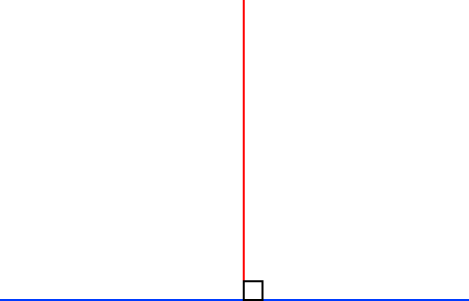# Definition of OrthogonalTwo lines are orthogonal if they are at right angles to each other.

For example, the $x$ and $y$-axes are orthogonal, and the sides of a right-angled triangle (other than the hypotenuse) are orthogonal.

Another word for "orthogonal" is "perpendicular".

There are also concepts of orthogonality for three-dimensional shapes and in other fields of mathematics.

### Description

The aim of this dictionary is to provide definitions to common mathematical terms. Students learn a new math skill every week at school, sometimes just before they start a new skill, if they want to look at what a specific term means, this is where this dictionary will become handy and a go-to guide for a student.

### Audience

Year 1 to Year 12 students

### Learning Objectives

Learn common math terms starting with letter O

Author: Subject Coach
You must be logged in as Student to ask a Question.